UIHistories Project: A History of the University of Illinois by Kalev Leetaru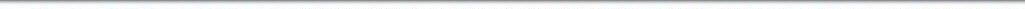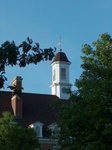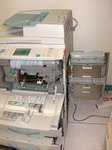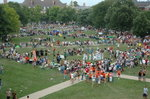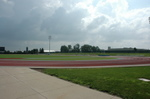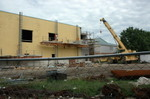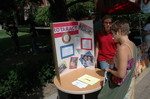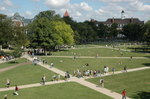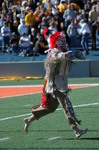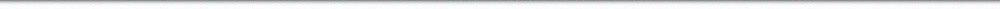N A V I G A T I O N D I G I T A L L I B R A R Y

## Repository: UIHistories Project: Board of Trustees Minutes - 1896 [PAGE 39]

Caption: Board of Trustees Minutes - 1896
This is a reduced-resolution page image for fast online browsing.

< Previous Page [Displaying Page 39 of 371] Next Page >
[VIEW ALL PAGE THUMBNAILS]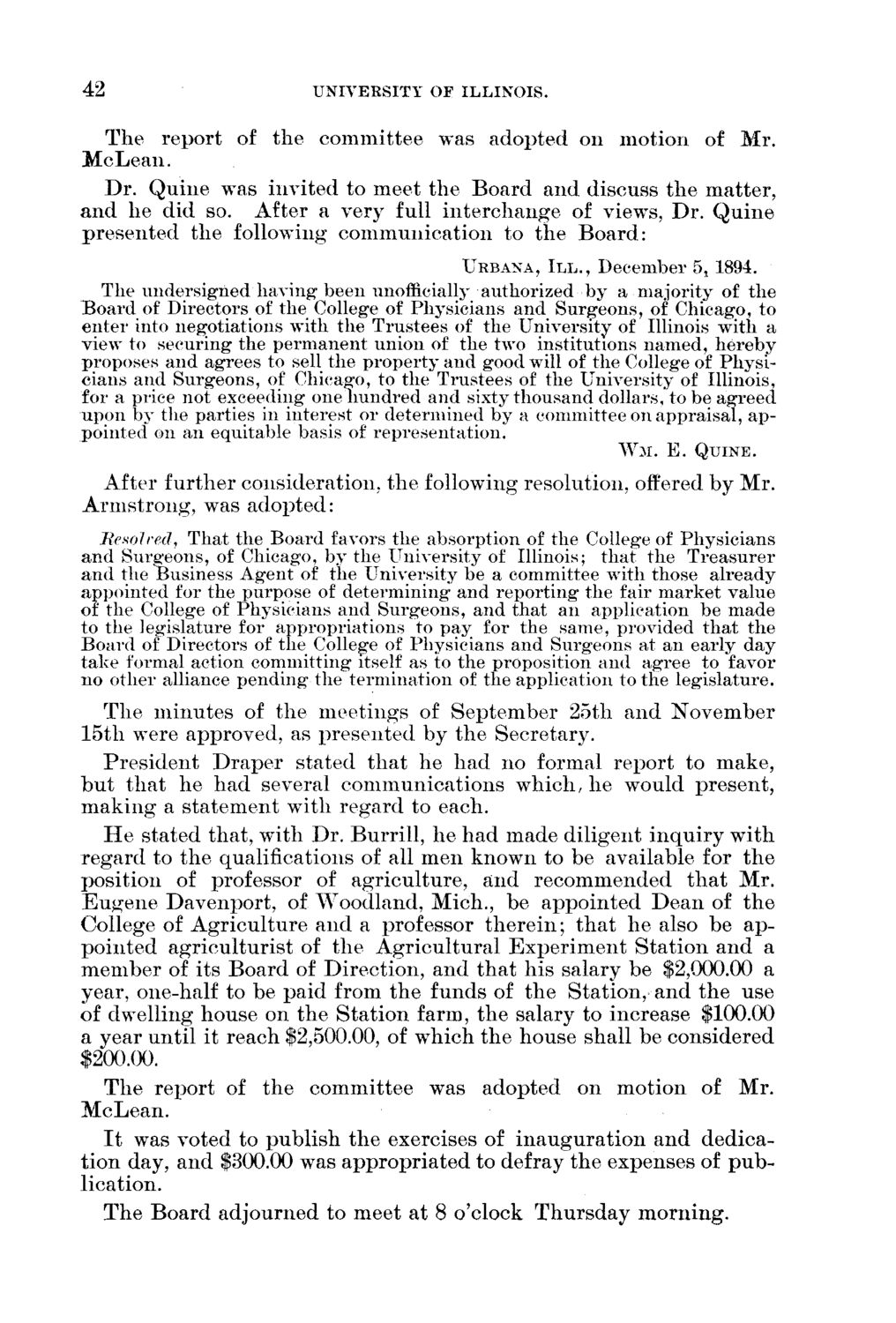### EXTRACTED TEXT FROM PAGE:

42

UNIVERSITY OF ILLINOIS.

T h e report of t h e c o m m i t t e e was a d o p t e d on m o t i o n of M r . McLean. D r . Q u i n e was invited to meet t h e B o a r d a n d discuss t h e m a t t e r , a n d he did so. After a very full i n t e r c h a n g e of viewTs, D r . Q u i n e p r e s e n t e d t h e following c o m m u n i c a t i o n to t h e B o a r d :

URBANA, I I I . , December 5* 1894.

The undersigned having1 been unofficially authorized by a majority of the Board of Directors of the College of Physicians and Surgeons, of Chicago, to enter into negotiations with the Trustees of the University of Illinois with a view to securing the permanent union of the two institutions named, hereby proposes and agrees to sell the property and good wTill of the College of Physicians and Surgeons, of Chicago, to the Trustees of the University of Illinois, for a price not exceeding one hundred and sixty thousand dollars, to be agreed upon by the parties in interest or determined by a committee on appraisal, appointed on an equitable basis of representation.

W M . E. QUINE.

After f u r t h e r consideration, t h e following resolution, offered b y M r . A r m s t r o n g , was a d o p t e d : Resolved, That the Board favors the absorption of the College of Physicians and Surgeons, of Chicago, by the University of Illinois; that the Treasurer and the Business Agent of the University be a committee with those already appointed for the purpose of determining and reporting the fair market value of the College of Physicians and Surgeons, and that an application be made to the legislature for appropriations to pay for the same, provided that the Board of Directors of the College of Physicians and Surgeons at an early day take formal action committing itself as to the proposition and agree to favor no other alliance pending the termination of the application to the legislature. T h e m i n u t e s of t h e m e e t i n g s of S e p t e m b e r 25th a n d N o v e m b e r 15th wTere approved, as p r e s e n t e d by t h e Secretary. P r e s i d e n t D r a p e r stated t h a t h e h a d no formal report to m a k e , b u t t h a t h e h a d several c o m m u n i c a t i o n s which/ h e would p r e s e n t , m a k i n g a s t a t e m e n t with r e g a r d to each. H e stated that, w4th D r . B u r r i l l , h e h a d made diligent i n q u i r y w i t h r e g a r d to t h e qualifications of all m e n k n o w n to be available for t h e position of professor of a g r i c u l t u r e , a n d r e c o m m e n d e d t h a t M r . E u g e n e D a v e n p o r t , of W o o d l a n d , Mich., be a p p o i n t e d D e a n of t h e College of A g r i c u l t u r e a n d a professor t h e r e i n ; t h a t h e also be a p p o i n t e d a g r i c u l t u r i s t of t h e A g r i c u l t u r a l E x p e r i m e n t S t a t i o n a n d a m e m b e r of its B o a r d of D i r e c t i o n , a n d t h a t h i s salary be \$2,000.00 a year, one-half to be p a i d from t h e funds of t h e S t a t i o n , a n d t h e use of dwelling house on t h e S t a t i o n farm, t h e salary to increase \$100.00 a year u n t i l it reach \$2,500.00, of w h i c h t h e house shall be considered \$200.00. T h e report of t h e c o m m i t t e e was adopted on m o t i o n of M r . McLean. I t was voted to p u b l i s h t h e exercises of i n a u g u r a t i o n a n d dedicat i o n day, a n d \$300.00 was a p p r o p r i a t e d to defray t h e expenses of p u b lication. T h e B o a r d adjourned to meet at 8 o'clock T h u r s d a y m o r n i n g .